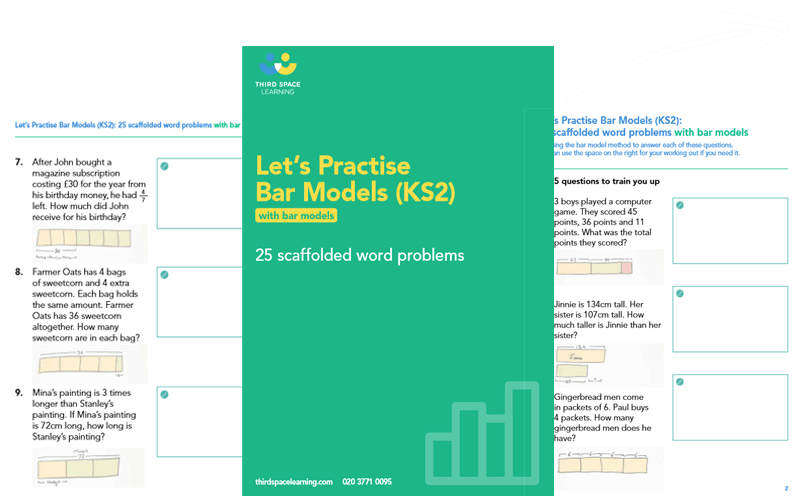Teaching support from the UK’s largest provider of in-school maths tuitionone to one lessonsschools supported

Built by teachers for teachers

In-school online one to one maths tuition developed by maths teachers and pedagogy experts

Find out more

Hundreds of FREE online maths resources!

Daily activities, ready-to-go lesson slides, SATs revision packs, video CPD and more!Explore all resources
Maths Mastery

Built by teachers for teachers

In-school online one to one maths tuition developed by maths teachers and pedagogy experts

Find out more

# The Fraction Bar Model: How To Teach Fractions Using Bar Models

The fraction bar model is a great way to introduce fractions to your young maths learners. Fractions can be a tricky concept for students to grasp and the visual representations of the bar model method can really help.Let's Practise Bar Model Word Problems

Download this worksheet and learn how to use bar models to answer a wide variety of maths problems.Download Free Now!

### What is the fraction bar model?

What is a bar model and how can it be used to teach fractions? Bar models are a ‘maths mastery’ technique and part of the concrete pictorial abstract approach of teaching mathematical concepts.

The fraction bar model uses a whole bar broken up into equal parts, a part whole model, to illustrate fractions of a whole. For example, a whole bar broken up into eight equal parts would be used to demonstrate eighths, and that eight eighths make up one whole.

A fraction wall uses a series of bar models to illustrate fractions and how different fractions relate to a whole.

### When do children learn fraction bar modelling?

According to the national curriculum, children will begin learning simple fractions, such as halves and quarters in Key Stage 1. As children progress into Key Stage 2, they will be introduced to increasingly complex maths problems using fractions where a bar model could help.

### Why teach bar model fractions?

Bar modelling is a great way to support childrens’ understanding of fractions and problem solving. Bar models can be used to teach both unit fractions and non-unit fractions. Bar models help children to visualise the maths in front of them, organise their thoughts and solve problems.

Bar modelling can also be used to teach number bonds, place value, addition, subtraction, percentage, solve word problems and for bar model multiplication and division.

Read more: Year 6 word problems

### How to draw a fraction bar model

Drawing a fraction bar model is simple:

• Draw a rectangle, it does not matter what size.
• Look at the denominator of the fraction you wish to represent.
• Divide the rectangle into the same number of equal parts as the denominator of the fraction.
• Shade in the same number of pieces as the numerator of the fraction.

### Finding fractions using the fraction bar model

Bar modelling can be used to help children  find fractions of an amount, for example ⅕ of 25. Instead of starting with the fraction, students start with the whole number which they then must divide into fifths.

The bar model helps to illustrate that five equal parts are added together to make the whole.

### Improper fractions and the fraction bar model

Improper fractions are fractions that have a larger numerator than the denominator. Children will often be asked to convert improper fractions into mixed numbers. In this case, bar modelling can be very useful.

A mixed number is a whole number combined with a fraction, for example, 3 \frac{2}{3}. This mixed number and its equivalent improper fraction can be represented using the fraction bar model.

### Equivalent fractions and the fraction bar model

Some maths problems may require children to identify equivalent fractions. Bar models can make this a lot easier as it removes the abstract and instead uses a concrete visualisation, enabling children to compare visuals and identify equivalent fractions.

### More fractions and bar models resources

Third Space Learning’s maths hub provides a wide range of primary resources for teaching fractions and bar models. Our online one-to-one tuition also uses bar models to help children  to gain a deep understanding of mathematical concepts.

Do you have pupils who need extra support in maths?
Every week Third Space Learning’s maths specialist tutors support thousands of pupils across hundreds of schools with weekly online 1-to-1 lessons and maths interventions designed to plug gaps and boost progress.

Since 2013 we’ve helped over 150,000 primary and secondary school pupils become more confident, able mathematicians. Learn more or request a personalised quote for your school to speak to us about your school’s needs and how we can help.

Subsidised one to one maths tutoring from the UK’s most affordable DfE-approved one to one tutoring provider.##### Vanessa Sipple-Asher
Third Space Learning
Content Team
Vanessa edits a lot of the content that you see on the Third Space Learning blog and once in a while, she gets the chance to write articles about maths education and how Third Space Learning can help students and teachers.

Related Articles

x#### Let's Practise Bar Model Word Problems

Download this worksheet and learn how to use bar models to answer a wide variety of maths problems.

#### Let's Practise Bar Model Word ProblemsDownload this worksheet and learn how to use bar models to answer a wide variety of maths problems.